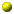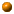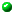Math 213a: Complex Analysis [Functions of One Complex Variable]
Fall 2003

If you find a mistake, omission, etc., please let me know by e-mail.

The orange balls mark our current location in the course, and the current problem set.Initial handout (DVI, PDF)
update 1.x.03: Matt B.'s office hours are Tuesdays, 1-2 in Sci Ctr 321d; section meets Thursday 5-6 in Sci Ctr 310. Both start next week (7 and 9 Oct).Concerning Ahlfors I.3, on ``linear transformations'' (usually called ``fractional linear transformations'' nowadays):

The Riemann sphere is the special case n=1, k=C of n-dimensional projective space over a field k, usually denoted by Pn(k). This consists of the lines (1-dimensional subspaces) of a k-vector space V of dimension n+1; in other words, Pn(k)=(V-{0}) / k*. The general point of Pn(k) is (x0:x1:x2:...:xn) where the coordinates xi are elements of k, not all zero, separated by colons rather than commas to emphasize that proportional (n+1)-tuples yield the same point in n-dimensional projective space. We may also write this space as P(V) when we do not want to commit ourselves to a choice of coordinates. A d-dimensional subspace of P(V) is the subset obtained from a (d+1)-dimensional subspace of V. It is thus itself a projective space, of dimension d. In particular, when d=n-1 we call the subspace a ``hyperplane''. The complement of a hyperplane is an ordinary affine n-dimensional space over k. [An ``affine space'' is a vector space without a choice of origin; for instance the Euclidean plane is an affine 2-dimensional space over R.]

The linear group GL(V)=GLn+1(k) acts on P(V)=Pn(k). The action is not faithful (unless k is the field of 2 elements): the kernel is the group of scalar matrices. We thus obtain an action on P(V) by the quotient group GL(V) / k*, usually known as the projective linear group PGL(V)=PGLn+1(k). It is a standard fact from linear algebra that GL(V) acts simply transitively on the ordered bases for V. Likewise, the affine linear group (the semidirect product of GL(V) with V, acting on the affine space associated to V) acts simply transitively on the ordered (n+1)-tuples of points of V not contained in an affine hyperplane. Finally, PGL(V) acts simply transitively on the ordered (n+2)-tuples of points of P(V) no n+1 of which are contained in a hyperplane. When n=1, this condition reduces to the requirement that the 1+2=3 points be distinct, and we recover the fact that PGL2(k) acts simply 3-transitively on the projective line over k.

We shall soon see that the fact that fractional linear transformations preserve angles between circles is a very special case of the fundamental result that analytic functions with nonzero derivative are conformal (angle-preserving).Warning: while inversion takes circles to circles, it does not in general take the center of a circle to the center of the inverted circle. In fact the stabilizer in PGL2(C) of a circle acts transitively on the circle's complement in P1(C), so all points not on the circle are equivalent to the center: a sequence of inversions can take a circle to itself but take its center to any desired point on the Riemann sphere as long as that point isn't on the circle!We did not require that a function of a complex variable be continuously differentiable to qualify as analytic. This was not an oversight: as Goursat showed, Cauchy's integral theorem and all its wonderful consequences can be proved without the hypothesis of continuous differentiability (which is then seen to be redundant). See Ahlfors, p. 88-89.One explanation for why Cauchy's integral formula is such a powerful tool is that it represents an arbitrary analytic function as a linear combination of functions 1/(z-a) from which it inherits the desirable properties of smoothness, Taylor series, etc. (The ``linear combination'' is actually an integral over z, but it has much the same effect.)

An easy generalization of Liouville's Theorem: if  f is an entire function such that f(z)=o(|z|M) as |z| goes to infinity, then f is a polynomial of degree less than M. By the Fundamental Theorem of Algebra, it follows that f can be written as a constant multiple of the product of fewer than M factors z-zj (the zj being the zeros of  f, with multiplicity). Later in the term we shall see that if  f satisfies the much weaker growth condition f(z)<<exp(|z|M) then f still has a product expansion ranging over zeros of  f, no more than RM+o(1) of which can fall in a large circle |z|<R (though the total number of zeros may be infinite in this setting).We saw that the Fourier expansion for an analytic function on the circle can be recovered from the formula for Laurent series. Likewise several other manifestation of Fourier analysis (notably, some cases of evaluation and inversion of one-dimensional Fourier transforms, and Poisson summation) can be obtained from contour integration when the functions in question extend to nice enough analytic functions of a complex variable.The argument principle (Ahlfors, Thm.20 on p.123, see also Thm.10 on p.103) has the following interpretation: if f has neither pole nor zero on gamma, then the winding number of f(gamma) around zero equals the difference between number of zeros and poles of f in the interior of gamma, weighted according to multiplicity and winding number. In particular, if there are no zeros or poles, the winding number of f(gamma) around the origin vanishes. The Fundamental Theorem of Algebra can now be recovered from the fact that if f is a polynomial of degree n, and gamma is a big enough circle, then f(gamma) winds n times around the origin.Principal values of integrals. Recall that the ``improper'' integral of a locally integrable function over R is defined as the limit of its integral over [-M,M'] as M and M' approach infinity independently. It may happen that the limit does not exist, but that the integrals over intervals [-M,M] do approach some finite limit; that limit is then called the principal value of the integral. For example, the integral over R of a locally integrable odd function always exists and equals zero. Likewise, if a function is locally integrable on an interval (a,b), except for a singularity at an internal point x, then its integral over (a,b) is defined to be the limit as e,e' approach zero of the integral over (a,b)-(x-e,x+e'); the principal value is the limit as e->0 of the integral over (a,b)-(x-e,x+e), which may exist even if the integral does not converge. Contour integration naturally yields evaluations of both kinds of principal-value integrals; notably, the integral over R of sin(x)/x can be evaluated (as Pi) in this way.The theorem that a limit of analytic functions that's uniform on compacta is itself analytic (Ahlfors, p.138) works -- with essentially the same proof -- for functions from and to arbitrary Riemann surfaces. (Uniformity makes sense, because the image of a compact set is itself compact, and so has a finite cover by neighborhoods identified with subsets of C, from which we inherit a definition of uniform convergence.) Weierstrass's formulation via uniformly convergent infinite sums (p.139), and termwise differentiation of such sums, require that the functions be complex-valued, though they can still be defined on an arbitrary Riemann surface. [But see problem 2 on the fifth problem set.]We noted that by taking z=1/2 in both sides of the partial-fraction expansion of (Pi/sin(Pi z))2 we can recover Euler's evaluation of zeta(2). More generally, for any k=2,4,6,8,... we can evaluate zeta(k) as a rational multiple of Pik by comparing Laurent expansions about z=0 (or Taylor expansions about z=1/2) of (Pi/sin(Pi z))2 and the sum over n of (1/(z-n))2. What do we get by expanding about z=1/4 instead? For a different take on these sums, see this recent article from the American Math. Monthly.Last year I taught a course on analytic number theory, for which I wrote some notes on the Hadamard product formula for an entire function of finite order. Here they are, in DVI and PDF. Likewise for my notes from the same course on the Gamma function (DVI, PDF).The proof in Ahlfors of the Riemann Mapping Theorem (IV.4.2, Thm.10, p.172ff.) is less mysterious than it may at first seem. Suppose the result is true. Then f'(z0) must be the largest value of |g'(z0)| over all functions g, univalent or not, taking Omega to the unit disc. Proof: consider the function on the unit disc taking z to f-1(g(z)).

For that matter, Ahlfors' definition of a ``normal family'', and the statement of Theorem 8 might not be what one would expect from the Arzela-Ascoli theorem. What Ahlfors is actually defining is the special case S=P1(C) of a normal family of analytic functions from Omega to a Riemann surface S. In general, a family of continuous functions on a metric space with a countable covering by compact subspaces is normal iff it is uniformly bounded and equicontinuous on compacta. When we're dealing with analytic functions, equicontinuity is implied by uniform boundedness of the derivative. When S=C, uniform boundedness of f implies uniform boundedness of f' by the Cauchy integral formula for f'. When S is a compact Riemann surface such as P1(C), uniform boundedness of f is automatic, and uniform boundedness of f' yields the f'/(1+|f|2) criterion. Note that Ahlfors might as well have allowed meromorphic functions in his definition, which would make the situation a bit clearer.

In addition to the tricks we've already seen for constructing conformal maps to the unit disc, here's how to conformally map the unit disc to a triangle: use the indefinite integral of (1-z/a)A-1(1-z/b)B-1(1-z/c)C-1dz, where a,b,c are on the unit circle and A,B,C are positive numbers with A+B+C=1. This takes a,b,c to the vertices of a triangle with angles Pi*a, Pi*b, Pi*c. What happens if one of A,B,C is taken to equal zero? [By following this map with a fractional linear transformation we may obtain any ``triangle'' bounded by arcs of three concurrent circles. ``Triangles'' bounded by arcs of three arbitrary circles will be treated when we discuss hypergeometric functions.]Since the space of harmonic functions on a region is invariant under conformal transformation, we can (and shall) consider harmonic functions also on Riemann surfaces that might not be equivalent to subsets of C.

The notation *du is a special case of the "Hodge star" acting on differential 1-forms on a region in a real inner-product space of dimension 2: *(a dx + b dy) = (a dy - b dx). In general, the Hodge star takes k-forms on n-space to (n-k)-forms.

As noted already, the fact that a function on a simply-connected region is harmonic if and only if it is the real part of an analytic function implies the remarkable fact that a harmonic function is automatically smooth (and in fact real-analytic). This is an example of a remarkable property of ``elliptic differential equations'', which holds also for harmonic functions in higher dimension. Note that already in dimension 2 a function satisfying the ``hyperbolic differential equation'' uxx-uyy=0, or equivalently urs=0 where (r,s)=(x+y,x-y), need not have any additional differentiability.

Formula (12) on p.181, giving a harmonic function PU(z) on the interior of the unit disc in terms of its boundary values U(z), has the following interpretation: let Sumnanzn be the Fourier series for U(z) on |z|=1 (in which an,a-n must be complex conjugates); then PU(z) is a0 plus twice the real part of Sumnanzn over positive n.

Much of our development of (sub)harmonic functions works just as well for regions in Rn for any dimension n. See for instance the last section of the seventh problem set.

Ahlfors' construction of a barrier function on the complement of a line segment (p.199) doesn't seem to quite work as stated, since the square root of (z-zeta0)/(z-zeta1) maps the complement of the line segment [zeta0zeta1] to a half-plane. However, using any positive exponent less than 1/2 instead of the square root is good enough.

The analysis leading up to Theorem 13 (p.202) is an example of a theme you'll likely see again in differential or algebraic geometry: it can be very useful to represent deRham cohomology (closed modulo exact differential forms on a manifold) by harmonic differentials.

The formula 3n-6 for the number of real parameters of a canonical region (Ahlfors, p.204) must be modified for n=2 and, of course, for n=1. We must add 1 and 3 respectively, due to the dimension of the group of automorphisms of the canonical region, which is respectively the ``infinite dihedral group'' of symmetries of a circle, and our old friend PSL2(R).

Another characterization of the solution u of the Dirichlet problem on some bounded region is that u is the continuously differentiable function on the region that minimizes I(u) the integral of (du/dx)2+(du/dy)2 among all functions with the same boundary values. (The physical interpretation of this integral is the energy stored in an electric field with potential function u.) Here it's easy to show that for each M the family of all continuously differentiable u with the same boundary values and I(u) bounded by M is equicontinuous, and nonempty if M is large enough. The hard part is showing that the limit of a sequence of functions u whose I(u) approach the infimum is again continuously differentiable, and then to show that it is in fact harmonic. The advantage of this method is that it applies readily to other regions with compact closure: integrate (du)^(*du) instead of ((du/dx)2+(du/dy)2) dx dy.At least in the edition that I've been working from, Ahlfors uses the word ``homotop'' (starting at VI.1.4). This is a rather old-fashioned [or Germanic] form of the word we know as ``homotopic''.

The Weierstrass preparation theorem may be stated as follows. Suppose f(z,w) is an analytic function of two variables on a neighborhood of (0,0) such that f(0,w) does not vanish identically but has a zero of order m>0 at w=0. Then there exists an integer k<=m and a punctured neighborhood of the origin in the z-plane on which the equation f(z,w)=0 has k solutions for each z, each analytic in z1/r for some positive integer r<=k.

The Wronskian of analytic functions f1,f2,...,fn is the zero function if and only if the fk are linearly dependent over C. Hence any n-dimensional space of analytic functions is the space of solutions of a unique regular linear differential equation of order n, namely Wrn+1(f1,f2,...,fn,w)/Wrn(f1,f2,...,fn)=0.

On a general Riemann surface S, a linear first-order differential equation looks like w'+pw=0 where p is a differential on S. A linear second-order diff.eq. is w''+pw'+qw=0, where p is almost a differential (it transforms a bit differently under coordinate changes) and q is a ``quadratic differential'', given in local coordinates by f(z)(dz)2 for some meromorphic function f. Likewise for linear differential equations of higher order.Handout on (lattices and) doubly periodic functions (DVI, PDF) corrected (typo in second display on p.11)
NB: The TeX command for the Weierstrass P-function is \wp .
The argument for Prop.3, part (ii), shows in general that for any compact Riemann surface S and any point p0 on S, the field F of rational functions on S is the fraction field of its subring A consisting of the functions with no poles except possibly p0, provided that A contains at least one nonconstant function. Then the determination of A suffices to reconstruct F.Zeroth problem set: Review of complex arithmetic (DVI, PDF)First problem set: inversive geometry and algebra (DVI, PDF)
corrected: parts (iii-iv) of #6 are about unitary matrices of unit determinantConcerning Problem 1(ii): the group in question is the orthogonal group O(n+1,1), acting on the vector space spanned by 1,x1,x2,...,xn,|x|2 whose elements a+b1x1+...+bnxn+c|x|2 of positive discriminant |b|2-4ac yield spheres in Rn. This ought to be much better known than it is.Second problem set: analytic functions, cont'd; Cauchy applications I (DVI, PDF)Third problem set: Differentials, residues, Laurent series (DVI, PDF)Concerning Problem 3: There are also elementary but ad-hoc derivations of these power series; see abel, catalan, catalan2 under ``one-page papers''.Fourth problem set: Contour integration II (DVI, PDF)Fifth problem set: Series of analytic functions; partial fractions and product formula (DVI, PDF)
corrected: in #4iii, a term L(f) was missing; in #5i, the sum should extend only over large real zeros xkSixth problem set: The Gamma function; univalent functions and normal families (DVI, PDF)Seventh problem set: Harmonic functions and the Dirichlet problem (DVI, PDF)My apologies for the bad Problem 5. :-(Eighth problem set: Harmonic functions and their uses, cont'd (DVI, PDF)
corrected: see problem 3 (incorrect conclusion) and problem 4 (missing hypothesis)Ninth problem set: Hypergeometric functions (DVI, PDF)